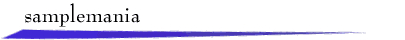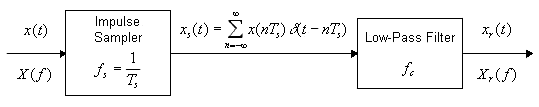A continous-time signal x(t) is sampled at a frequency of fs Hz to produce a sampled signal xs(t). We model xs(t) as an impulse train with the area of the nth impulse given by x(nTs ). An ideal low-pass filter with cutoff frequency fc Hz is used to obtain the reconstructed signal xr(t).

Suppose the highest-frequency component in x(t) is at frequency fm. Then the Sampling Theorem states that for fs > 2fm there is no loss of information in sampling. In this case, choosing fc in the range fm <fc <fs - fm gives xr(t) = x(t). These results can be understood by examining the Fourier transforms X(f), Xs(f), and Xr(f). If fs < 2fm and/or fc is chosen poorly, then xr(t) might not resemble x(t).

To explore sampling and reconstruction, select a signal or use the mouse to draw a signal x(t) in the window below. After a moment, the magnitude spectrum |X(f)| will appear. Then, enter a sampling frequency fs and click "Sample" to display the sampled signal and its magnitude spectrum. Finally, choose a cutoff frequency fc and click "Filter." The reconstructed signal and its magnitude spectrum will be shown.return to demonstrations page

 Applet by Steve Crutchfield.